Equivalent Resistance of Resistors (Parallel)

# Our Objective

To determine the equivalent resistance of two resistors when connected in parallel.

# The Theory

Before we start learning about parallel circuits, we need to know what electrical circuits are and about  the types of circuits!

## Electrical Circuit

An electric circuit is simply a closed loop through which charges can continuously move. An electric circuit basically contains a source of electricity, a load resistance, a switch or a key for turning the circuit on or off at one's convenience.

The diagrammatic representation of an electric circuit is called the circuit diagram.## Types of circuits

When two or more resistors are connected in such a way that one end of one resistance is connected to the starting end of the other, then the circuit is called Series Circuit.

Similarly, if the starting ends of two resistors are joined to a point, and the terminal ends of the two are combined and given connection to a source of electricity, those circuits are called Parallel Circuit.

Unlike in the series circuit, the current in each branch of a parallel circuit will be different. If one branch is broken, current will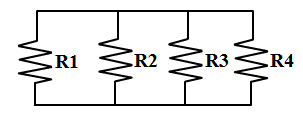continue flowing to the other branches.

At our homes all the electronic appliances are connected in parallel with each other.  This means they all get the full mains voltage, so that we can turn on the TV without having to turn on the microwave as well.

## Before calculating the equivalent resistance, we need to know what resistance is?

Electrical resistance shows how much energy you need when you move the charges/current through your devices. If you need lots of energy, then the resistance required is also high.

Ie., the property of a conductor to oppose the flow of charges through it is called its resistance.

It is measured as resistance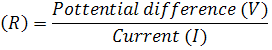ie.,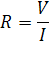All metals are good conductors so all metal wires have a relatively low resistance.

The longer a piece of wire the higher is its resistance.  Double the length means double the resistance.

ie.,The resistance of a piece of wire also depends on its thickness / cross-sectional area A of the conductor.  Double the area means half the resistance.  A thin wire has a higher resistance than the same thick piece.

ie.,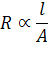Finally, resistance of a piece of wire depends on its temperature also.  The higher the temperature the higher is the resistance.

## Calculating the effective resistance/total resistance of parallel circuits:

Calculating the equivalent resistance of two or more resistors in parallel is a little more complicated than calculating the total resistance of two or more resistors in series.

Given two resistors, R1 and R2, in parallel, the equivalent resistance, Rt, is: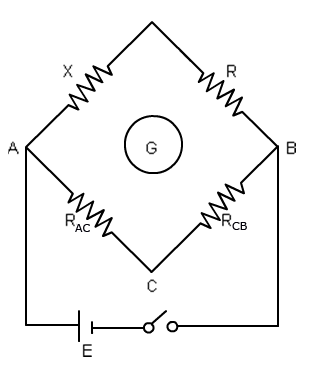That is, for a set of parallel resistors, the reciprocal of their equivalent

resistance equals the sum of the reciprocals of their individual resistances.

Thus, resistance decreases in parallel combination.

Using the formula is not too difficult.  Simply substitute  your values for the resistances and then add up the fractions. To find the equivalent resistance (Rt), you need to upside down your result.

Here, we used Meter Bridge to calculate the effective resistance accurately.
The Meter Bridge is a device for measurement of resistance using the principle of Wheatstone Network.

So, go ahead and try out the experiment as per the procedures and find the equivalent resistance!!